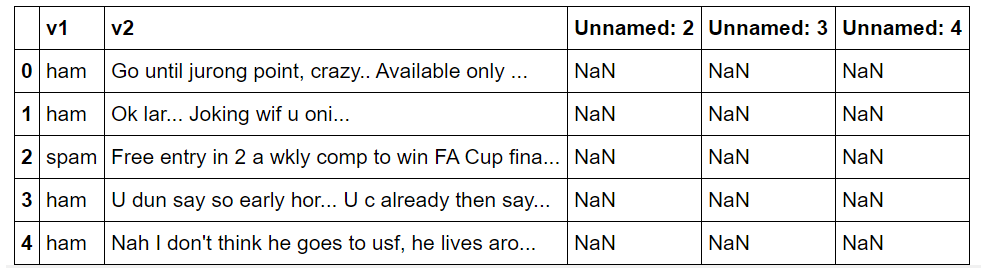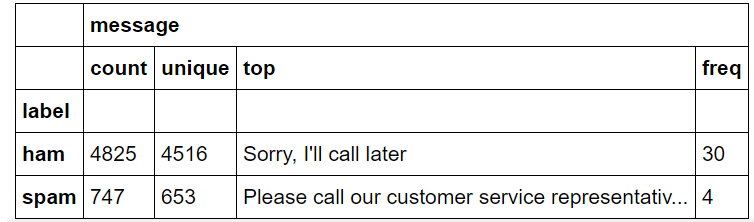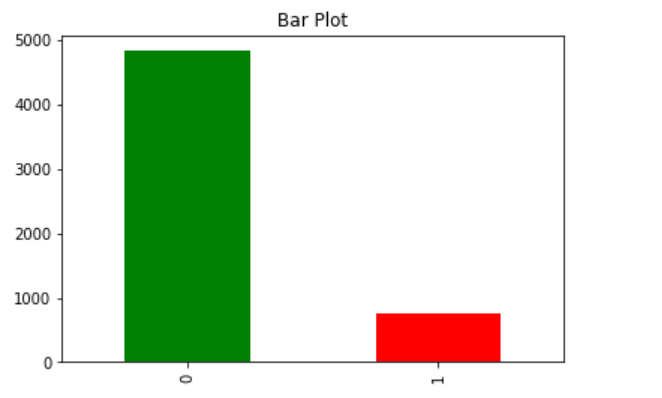# Build SMS Spam Classification Model using Naive Bayes & Random ForestBuild SMS Spam Classification Model using Naive Bayes & Random Forest. Building SMS Spam Classification using Python and Pandas. If you are into data science and looking for starter projects then the SMS Spam classification Project is one of those you should work upon! In this tutorial, we would go step by step from importing libraries to full model prediction and lately measuring the accuracy of the model.

If you are into data science and looking for starter projects then the SMS Spam classification Project is one of those you should work upon! In this tutorial, we would go step by step from importing libraries to full model prediction and lately measuring the accuracy of the model.

A good text classifier is a classifier that efficiently categorizes large sets of text documents in a reasonable time frame and with acceptable accuracy, and that provides classification rules that are humanly readable for possible fine-tuning. If the training of the classifier is also quick, this could become in some application domains a good asset for the classifier. Many techniques and algorithms for automatic text categorization have been devised.

The text classification task can be defined as assigning category labels to new documents based on the knowledge gained in a classification system at the training stage. In the training phase, we are given a set of documents with class labels attached, and a classification system is built using a learning method. Classification is an important task in both data mining and machine learning communities, however, most of the learning approaches in text categorization are coming from machine learning research.

## Building SMS Spam Classification using Python, Pandas

For this project, I would be using Google Colab, but you can use python Notebook also for the same purpose.

Importing of Libraries

First, we would import the required libraries such as pandas, matplotlib, numpy, sklearn

``````import numpy as np
import pandas as pd
import matplotlib.pyplot as plt
import seaborn as sns
import scipy as sp
from sklearn import feature_extraction, model_selection, naive_bayes, metrics, svm
from sklearn.ensemble import RandomForestClassifier
from sklearn.model_selection import train_test_split
from sklearn.metrics import precision_recall_fscore_support as score
%matplotlib inline
drive.mount('/content/drive')``````

Note: the last line of the code snippet can be removed if you are not using Google Colab. This last line is for mounting my Google Drive over Google Colab so that I can use the dataset present in my drive.

Importing the dataset

I would be uploading the dataset in my GitHub repo which can be found here.

``dataset = pd.read_csv("/content/drive/My Drive/SMS_Spam_Classification/spam.csv", encoding='latin-1')``

Now as we have imported the dataset, let's see if we have imported the dataset incorrect format or not by using head() function.

``dataset.head()``From the above dataset snippet, I see that we have the column names which we don't require! Thus now comes the task of cleaning and reformatting the data for us to use it to build our model.

Data Cleaning & Exploration

Now we have to remove unnamed columns. To do so we would use the drop function.

``````#removing unnamed columns
dataset = dataset.drop('Unnamed: 2', 1)
dataset = dataset.drop('Unnamed: 3', 1)
dataset = dataset.drop('Unnamed: 4', 1)``````

Now, the next task is to rename the columns v1 and v2 to label and message respectively!

``dataset = dataset.rename(columns = {'v1':'label','v2':'message'})``

Now, additionally (its an optional step but its always good to do some data exploration also :P )

``dataset.groupby('label').describe()``Next thing we want to know how many messages are ham and how many messages are spam in our dataset. For that:

``````count_Class=pd.value_counts(dataset["label"], sort= True)
count_Class.plot(kind = 'bar',color = ["green","red"])
plt.title('Bar Plot')
plt.show();``````

Explanation: Here we set the sort = True and use the value_counts method of Pandas. This code would make a bar plot of green and red color respectively for spam and not spam classes.

The output you might be getting would be similar to this:We see that we have a lot of ham messages whereas less spam messages. In this tutorial, we would go on forward with this dataset only without augmenting it (no oversampling/under sampling) I would do here.

## Data Science Projects | Data Science | Machine Learning | Python

Practice your skills in Data Science with Python, by learning and then trying all these hands-on, interactive projects, that I have posted for you.

## Data Science Projects | Data Science | Machine Learning | Python

Practice your skills in Data Science with Python, by learning and then trying all these hands-on, interactive projects, that I have posted for you.

## Data Science Projects | Data Science | Machine Learning | Python

Practice your skills in Data Science with Python, by learning and then trying all these hands-on, interactive projects, that I have posted for you.

## Data Science Projects | Data Science | Machine Learning | Python

Practice your skills in Data Science with Python, by learning and then trying all these hands-on, interactive projects, that I have posted for you.

## Data Science Projects | Data Science | Machine Learning | Python

Practice your skills in Data Science with Python, by learning and then trying all these hands-on, interactive projects, that I have posted for you.